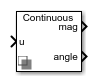# Sequence Analyzer

Calculate the magnitude and phase of the positive, negative, or zero sequence of a three-phase signal

Since R2019b

•Libraries:
Simscape / Electrical / Control / Measurements

## Description

The Sequence Analyzer block takes a three-phase signal (usually voltage) as the input and outputs the magnitude and phase of the positive, negative, or zero sequence.

The input signals are first converted to phasor representation based on the base frequency and harmonic specified in the block mask. The block then transforms the phasor into a sequence of components..

You can represent the zero, positive, and negative sequence components with V0, V1, V2 in phasor representation; Va, Vb, Vc are the voltage of three phases. The sequence components are computed as:

`$\left[\begin{array}{c}{V}_{0}\\ {V}_{1}\\ {V}_{2}\end{array}\right]=\frac{1}{3}\left[\begin{array}{ccc}1& 1& 1\\ 1& a& {a}^{2}\\ 1& {a}^{2}& {a}^{2}\end{array}\right]\left[\begin{array}{c}{V}_{a}\\ {V}_{b}\\ {V}_{c}\end{array}\right]$`

where $a={e}^{j\frac{2}{3}\pi }$.

## Limitations

• Because the computation of the three-phase signal requires integration over a full cycle, the output of the sequence analyzer is not accurate during the first period.

• If the base frequency or the harmonic change during the simulation, the output signal is not accurate.

## Ports

### Input

expand all

Periodic input signal, specified as a vector of three elements.

### Output

expand all

Magnitude of the positive, negative, or zero sequence. Depending on the harmonic numbers, this output is a scalar or a vector.

Phase shift, in degrees, of the positive, negative, or zero sequence. Depending on the harmonic numbers, this output is a scalar or a vector.

## Parameters

expand all

Base frequency of the input signal in Hz.

Scalar or vector of the harmonic numbers. `1` stands for base frequency and `2` is the second harmonic.

Select whether the magnitude and phase of the sequence is positive, negative or zero.

Time between consecutive block executions. During execution, the block produces outputs and, if appropriate, updates its internal state. For more information, see What Is Sample Time? and Specify Sample Time.

For inherited discrete-time operation, specify `-1`. For discrete-time operation, specify a positive integer. For continuous-time operation, specify `0`.

If this block is in a masked subsystem, or other variant subsystem that allows you to switch between continuous operation and discrete operation, promote the sample time parameter. Promoting the sample time parameter ensures correct switching between the continuous and discrete implementations of the block. For more information, see Promote Block Parameters on a Mask.

## Version History

Introduced in R2019b